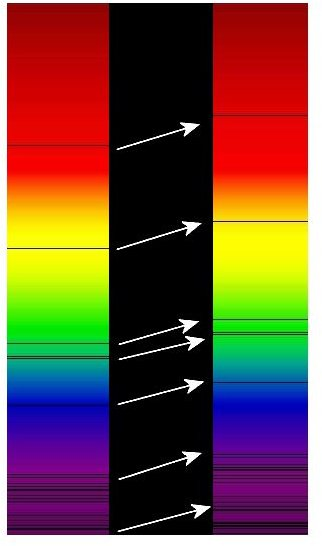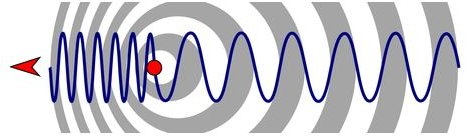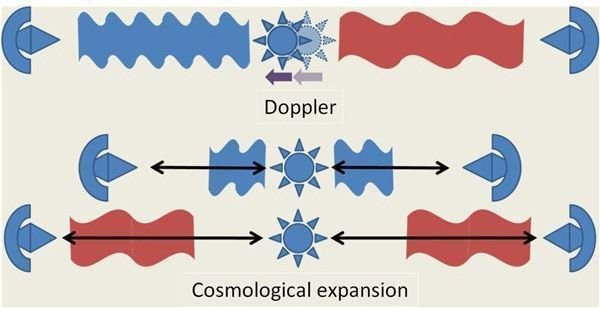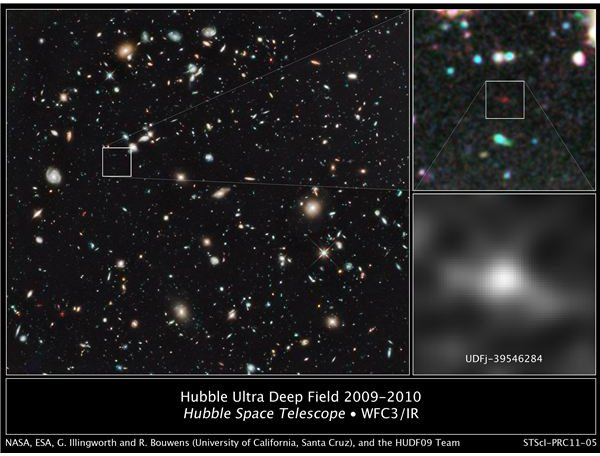# Defining Redshift in Astronomy

## What is a Redshift and How to Observe itThe phenomenon in which the observed light has a longer wavelength compared to the wavelength of the emitted light is called redshift. Let's assume that a light source emits light of wavelength λ0. If the observer sees this light with a wavelength of λ where λ>λ0, then we have a redshift. The opposite phenomenon in which the wavelength appears shorter to the observer is called blueshift.

The measure of this increase in wavelength is symbolized as z and can be calculated by the following equation:

z = (λο / λe) – 1

λο: is the wavelength of the observed light

λe: is the wavelength of the emitted light

The positive z value denotes a redshift, while a negative z value denotes a blueshift.

Astronomical redshift can be observed due to three phenomena:

a) The Doppler effect caused by the relative motion betwwen the source and the observer,

b) The effect of high density gravitational fields and

c) The expansion of the Universe still occurring after the Big Bang.

At the left of the image above, we can see the absorption lines of the Sun in the optical spectrum, as they are compared to the absorption lines of a galaxy supercluster (BAS11) at the right. The redshift is evident.

## Redshift Due to the Doppler EffectThe Doppler effect was discovered and named after Christian Doppler who realized that the emitted wavelength may differ from the observed wavelength due to the relative motion between the source and the observer.

An everyday example is the sound of the ambulance siren. The sound is high-pitched as the ambulance approaches and then becomes fainter as the ambulance is moving away

The non-relativistic equation of the Doppler effect (velocity – v less than 10% of light speed) is:

z = (λο – λe) / λe = v/c

v: radial velocity between the source and the observer

c : speed of light (~299,792 km/s)

## Redshift Due to Gravitation

Einstein's general theory of relativity predicts that the passing of light or any kind of electromagnetic wave near a gravitational field, causes a redshift. In simple terms, as the photon passes through a very large gravitational field, it looses energy and therefore increases its wavelength (equally decreasing frequency). Einstein used general relativity to predict that the deflection of light caused by the Sun's gravity near the edge of the star is 1.75 arc seconds. The value agrees with the experimental data.

However, the redshift caused by the gravitational field of the Sun is very small compared to the ones caused by extremely massive stars and black holes.

## Redshift due to Cosmological Expansion

After the Big Bang, a phenomenon known as the cosmological expansion of the Universe took place. This phenomenon is quite evident when distant objects (beyond our local group of galaxies) are observed and it causes the stretching of space and time that also affects light (theory of general relativity) . Due to this stretching, the wavelength of the emitted light increases and therefore becomes redshifted.A measure of the cosmological expansion is the Hubble constant H0, which is equal to 71 km/sec/Mpc. The value was determined after several observations made by the Wilkinson Microwave Anisotropy Probe (WMAP). Since the Universe is still expanding, this value will change over time.

## The Most Distant Object Ever Seen

As mentioned, the astronomical redshift observed can originate from the Doppler effect, the existence of gravitational fields and the cosmological expansion of the Universe. Depending on the distance and the existence of large masses nearby, one or more of these mechanisms may become more dominant in calculating z-redshifts. The general rule is that higher z values are due to the cosmological expansion.

The image below was taken by the Hubble Telescope and shows the most distant object ever seen. It is a proto-galaxy, 13.2 billion light-years away, with less than 1% of the size of Milky Way.Since light has been traveling for 13.2 billion years, we currently observe the condition of the galaxy back into the past. The z-redshift of this object is approximately 10.

## Sources:

• Astronomical Redshift by asterism.org: https://www.asterism.org/tutorials/tut29-1.htm
• "What is the Doppler effect?" by qrg.northwestern.edu: https://www.qrg.northwestern.edu/projects/vss/docs/communications/3-what-is-the-doppler-effect.html
• Doppler Effect by scienceworld.wolfram.com
• "The most distant object ever seen" by smartplanet.com

Image Credits:

• Redshift: Wikimedia Commons
• Diagram Showing the Change of Wavelength due to the Doppler Effect: Wikimedia Commons
• Redshift due to Doppler Effect and Cosmological Expansion: Wikimedia Commons
• nasaimages.org: The Most Distant Object Ever Seen